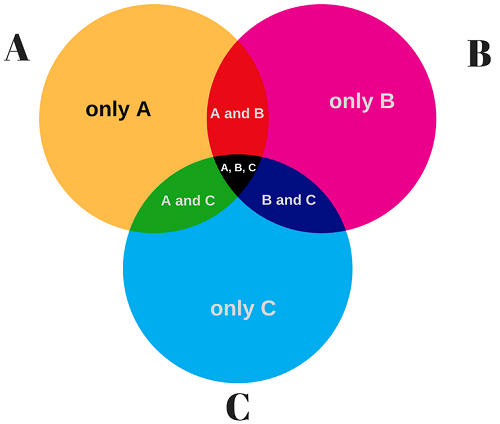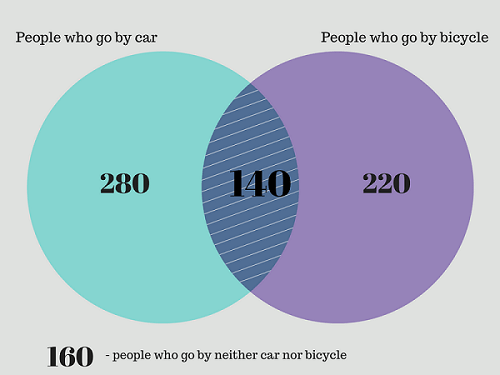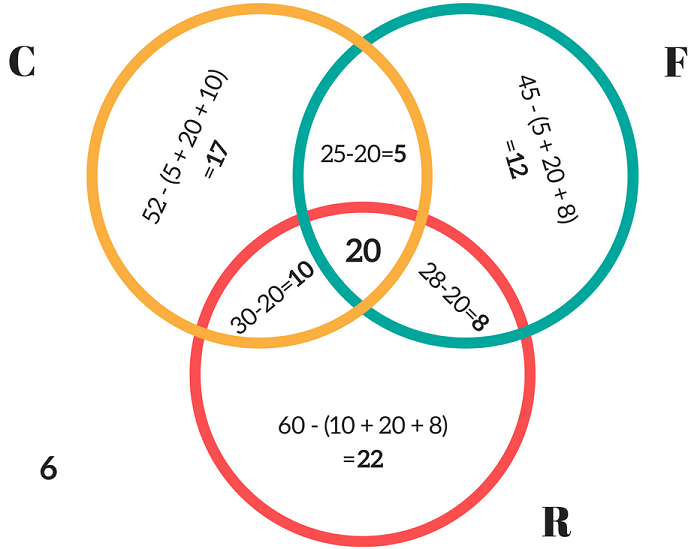# Venn Diagram Examples, Problems and Solutions

The best way to explain how the Venn diagram works and what its formulas show is to give 2 or 3 circles Venn diagram examples and problems with solutions.

Problem-solving using Venn diagram is a widely used approach in many areas such as statistics, data science, business, set theory, math, logic and etc.

• What is Venn diagram? Definition and meaning.
• Venn diagram formula with an explanation.
• Examples of 2 and 3 sets Venn diagrams: practice problems with solutions, questions, and answers.
• Simple 4 circles Venn diagram with word problems.
• Compare and contrast Venn diagram example.

Let’s define it:

A Venn Diagram is an illustration that shows logical relationships between two or more sets (grouping items). Venn diagram uses circles (both overlapping and nonoverlapping) or other shapes.

Commonly, Venn diagrams show how given items are similar and different.

Despite Venn diagram with 2 or 3 circles are the most common type, there are also many diagrams with a larger number of circles (5,6,7,8,10…). Theoretically, they can have unlimited circles.

Venn Diagram General Formula

n(A ∪ B) = n(A) + n(B) – n(A ∩ B)

Don’t worry, there is no need to remember this formula, once you grasp the meaning. Let’s see the explanation with an example.

This is a very simple Venn diagram example that shows the relationship between two overlapping sets X, Y.Where:

X – the number of items that belong to set A
Y – the number of items that belong to set B
Z – the number of items that belong to set A and B both

From the above Venn diagram, it is quite clear that

n(A) = x + z
n(B) = y + z
n(A ∩ B) = z
n(A ∪ B) = x +y+ z.

Now, let’s move forward and think about Venn Diagrams with 3 circles.Following the same logic, we can write the formula for 3 circles Venn diagram:

n(A ∪ B ∪ C) = n(A) + n(B) + n(C) – n(A ∩ B) – n(B ∩ C) – n(C ∩ A) + n(A ∩ B ∩ C)

Venn Diagram Examples (Problems with Solutions)

As we already know how the Venn diagram works, we are going to give some practical examples (problems with solutions) from the real life.

2 Circle Venn Diagram Examples (word problems):

Suppose that in a town, 800 people are selected by random types of sampling methods. 280 go to work by car only, 220 go to work by bicycle only and 140 use both ways – sometimes go with a car and sometimes with a bicycle.

Here are some important questions we will find the answers:

• How many people go to work by car only?
• How many people go to work by bicycle only?
• How many people go by neither car nor bicycle?
• How many people use at least one of both transportation types?
• How many people use only one of car or bicycle?

The following Venn diagram represents the data above:Now, we are going to answer our questions:

• Number of people who go to work by car only = 280
• Number of people who go to work by bicycle only = 220
• Number of people who go by neither car nor bicycle = 160
• Number of people who use at least one of both transportation types = n(only car) + n(only bicycle) + n(both car and bicycle) = 280 + 220 + 140 = 640
• Number of people who use only one of car or bicycle = 280 + 220 = 500

Note: The number of people who go by neither car nor bicycle (160) is illustrated outside of the circles. It is a common practice the number of items that belong to none of the studied sets, to be illustrated outside of the diagram circles.

We will deep further with a more complicated triple Venn diagram example.

3 Circle Venn Diagram Examples:

For the purposes of a marketing research, a survey of 1000 women is conducted in a town. The results show that 52 % liked watching comedies, 45% liked watching fantasy movies and 60% liked watching romantic movies. In addition, 25% liked watching comedy and fantasy both, 28% liked watching romantic and fantasy both and 30% liked watching comedy and romantic movies both. 6% liked watching none of these movie genres.

Here are our questions we should find the answer:

• How many women like watching all the three movie genres?
• Find the number of women who like watching only one of the three genres.
• Find the number of women who like watching at least two of the given genres.

Let’s represent the data above in a more digestible way using the Venn diagram formula elements:

• n(C) = percentage of women who like watching comedy = 52%
• n(F) = percentage of women who like watching fantasy = 45%
• n(R)= percentage of women who like watching romantic movies= 60%
• n(C∩F) = 25%; n(F∩R) = 28%; n(C∩R) = 30%
• Since 6% like watching none of the given genres so, n (C ∪ F ∪ R) = 94%.

Now, we are going to apply the Venn diagram formula for 3 circles.

94% = 52% + 45% + 60% – 25% – 28% – 30% + n (C ∩ F ∩ R)

Solving this simple math equation, lead us to:

n (C ∩ F ∩ R) = 20%

It is a great time to make our Venn diagram related to the above situation (problem):See, the Venn diagram makes our situation much more clear!

From the Venn diagram example, we can answer our questions with ease.

• The number of women who like watching all the three genres = 20% of 1000 = 200.
• Number of women who like watching only one of the three genres = (17% + 12% + 22%) of 1000 = 510
• The number of women who like watching at least two of the given genres = (number of women who like watching only two of the genres) +(number of women who like watching all the three genres) = (10 + 5 + 8 + 20)% i.e. 43% of 1000 = 430.

As we mentioned above 2 and 3 circle diagrams are much more common for problem-solving in many areas such as business, statistics, data science and etc. However, 4 circle Venn diagram also has its place.

4 Circles Venn Diagram Example:

A set of students were asked to tell which sports they played in school.

The options are: Football, Hockey, Basketball, and Netball.

Here is the list of the results:

 Sport Name Football Robert, James, John, Mary, Jennifer, William Hockey Robert, William, Linda, Elizabeth, James Basketball William, Jayne, Linda, Daniel, Mary Netball Jessica, William, Linda, Elizabeth, Anthony, Mary None Dorothy

The next step is to draw a Venn diagram to show the data sets we have.It is very clear who plays which sports. As you see the diagram also include the student who does not play any sports (Dorothy) by putting her name outside of the 4 circles.

From the above Venn diagram examples, it is obvious that this graphical tool can help you a lot in representing a variety of data sets. Venn diagram also is among the most popular types of graphs for identifying similarities and differences.

Compare and Contrast Venn Diagram Example:

The following compare and contrast example of Venn diagram compares the features of birds and bats:Tools for creating Venn diagrams

It is quite easy to create Venn diagrams, especially when you have the right tool. Nowadays, one of the most popular way to create them is with the help of paid or free graphing software tools such as:

You can use Microsoft products such as:

• Word
• Excel
• PowerPoint

Some free mind mapping tools are also a good solution. Finally, you can simply use a sheet of paper or a whiteboard.

Conclusion:

The above 2, 3 and 4 circle Venn diagram examples aim to make you understand better the whole idea behind this diagrams.

As you see, the Venn diagram formula can help you to find solutions for a variety of problems and questions from the real life.

A Venn diagram is a simple but powerful way to represent the relationships between datasets. It makes understanding math, different types of data analysis, set theory and business information easier and more fun for you.

Besides of using Venn diagram examples for problem-solving and comparing, you can use them to present passion, talent, feelings, funny moments and etc.

Be it data science or real-world situations, Venn diagrams are a great weapon in your hand to deal with almost any kind of information.

If you need more chart examples, our posts fishbone diagram examples and what does scatter plot show might be of help.